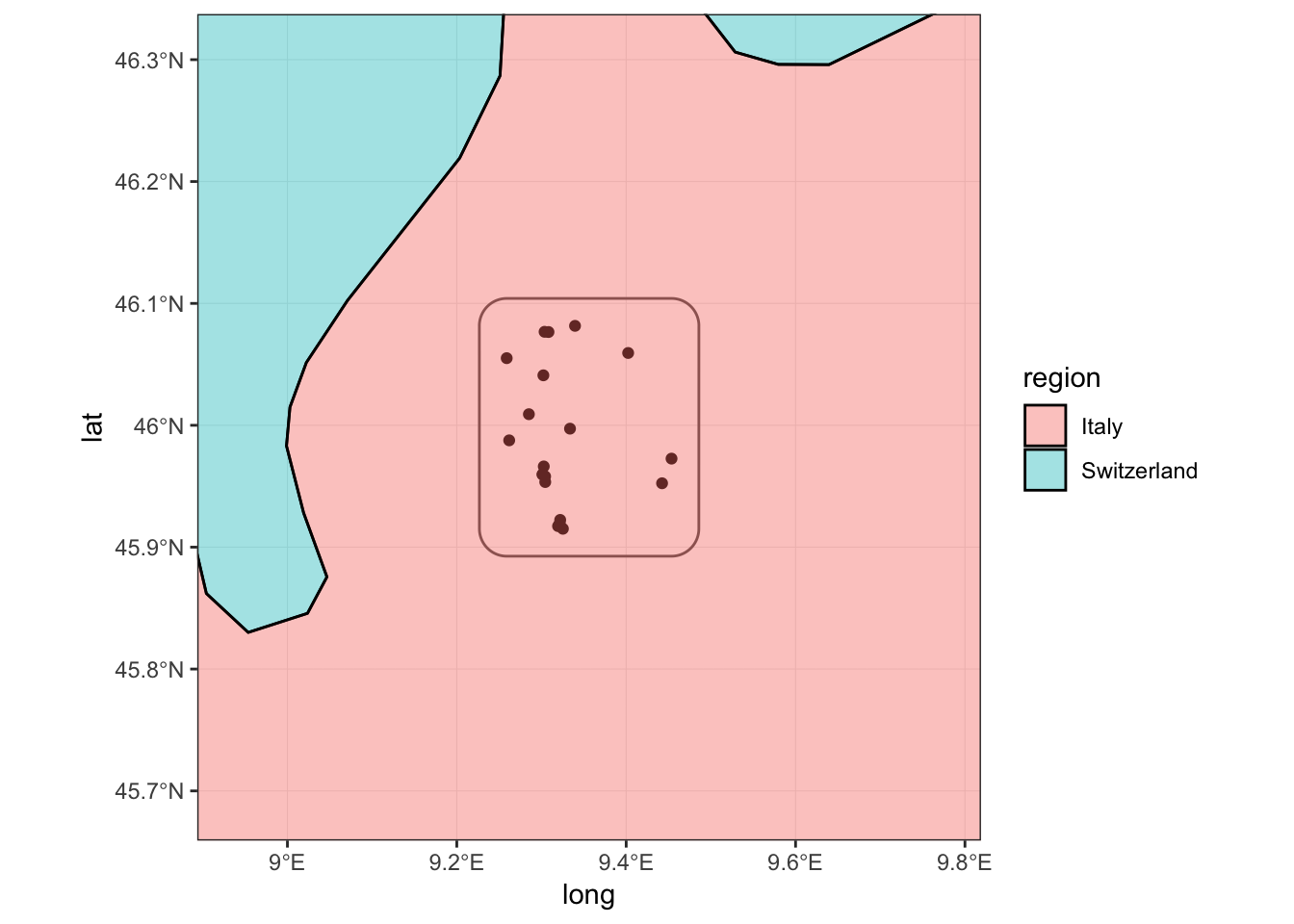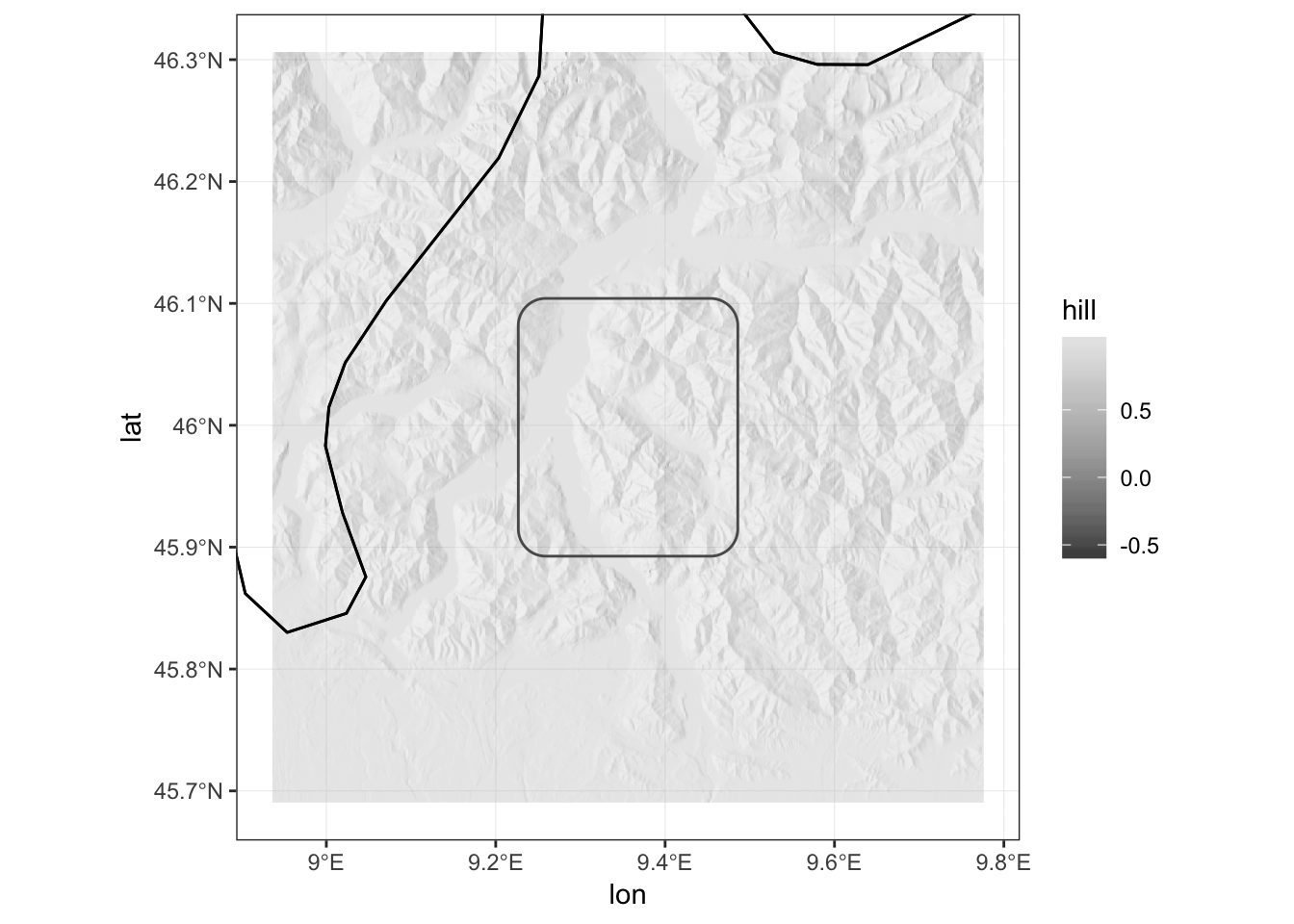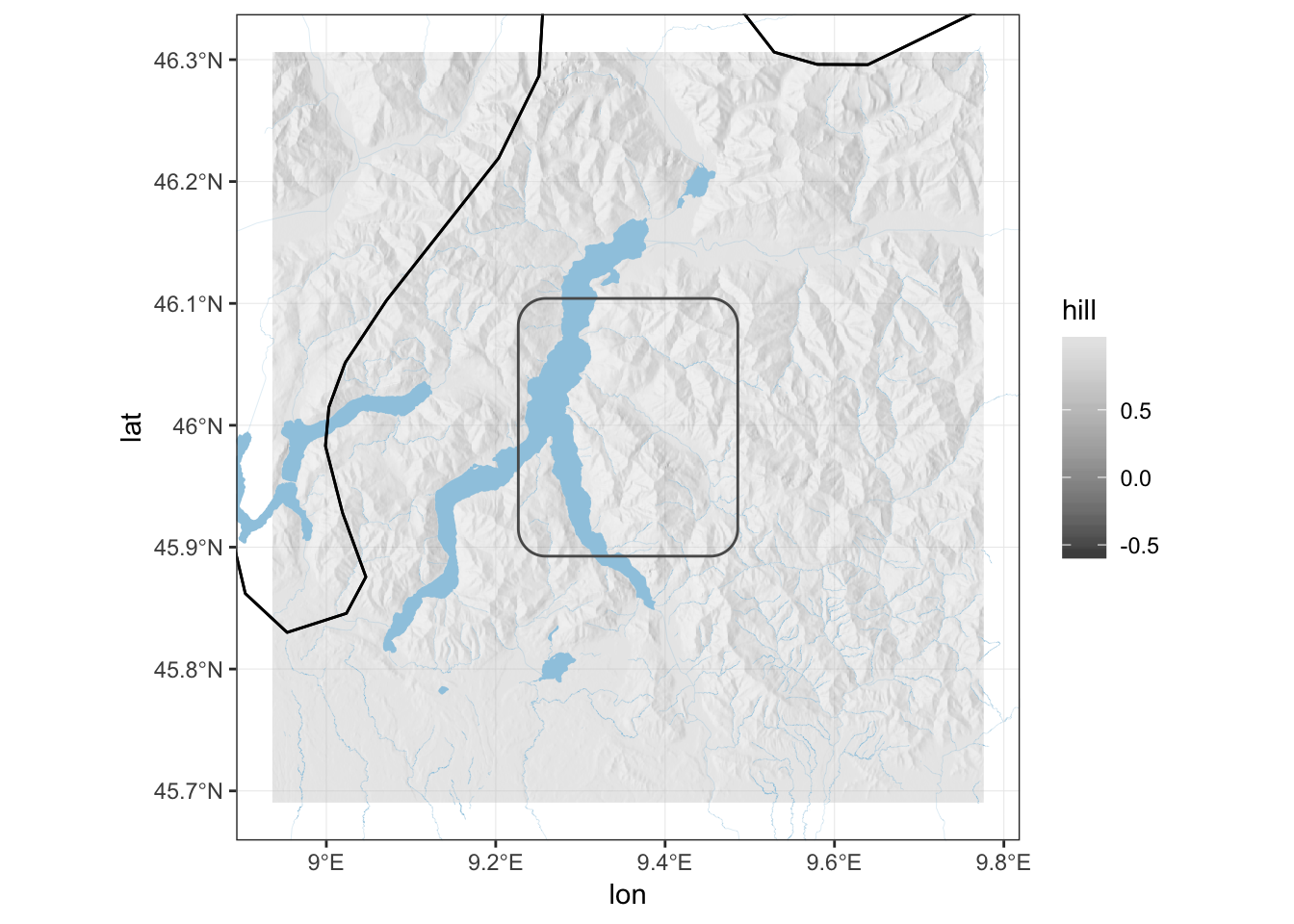# How to (quickly) enrich a map with natural and anthropic details

In this post I show how to enrich a ggplot map with data obtained from the Open Street Map (OSM) API. After adding elevation details to the map, I add water bodies and elements identifying human activity. To highlight the areas more densely inhabitated, I propose to use a density-based clustering algorithm of OSM features.

## Data

Let’s first start with some data: a few points somewhere in Italy.

my_points.df <-
data.frame(lon = c(9.442366, 9.30222, 9.304297, 9.322077,
9.304432, 9.302683, 9.321543, 9.301541, 9.402329, 9.285115, 9.319564,
9.33959, 9.33367, 9.261872, 9.308274, 9.3011, 9.45355, 9.32527,
9.25892, 9.303647),
lat = c(45.952427, 46.041, 45.958035, 45.922367,
45.953438, 45.966248, 45.919487, 45.959721, 46.05933, 46.009094,
45.917286, 46.081585, 45.997231, 45.98764, 46.076529, 45.959641,
45.972649, 45.9151, 46.055069, 46.07671))


I will use the great sf package so let’s create a sf spatial object with

library(sf)
my_points.sf <- st_as_sf(my_points.df, coords = c("lon", "lat"), crs = 4326)


crs = 4326 sets the Coordinate Reference System using an integer EPSG code. EPSG:4326 uses the World Geodetic System 1984 (WGS 84) that is used in GPS and its unit is the degree (not the meter!).

In this post I show how to enrich a ggplot map with data obtained from the Open Street Map (OSM) API. After adding elevation details to the map, I add water bodies and elements identifying human activity. To highlight the areas more densely inhabitated, I propose to use a density-based clustering algorithm of OSM features.

As a first step let’s define a bounding box that includes my points.

my_bbox <- c(xmin = min(my_points.df$lon), xmax = max(my_points.df$lon),
ymin = min(my_points.df$lat), ymax = max(my_points.df$lat))


Once I have set the points, I can create a polygon with the function sf::st_polygon(), which takes a two-column matrix for each polygon and create a simple feature (sf) object, and the function sf::st_geometry(), which takes a simple future object and get its geometry. Points need to be five because we always need to close our polygons! So the last (or 5th) row will have the same pair of coordinates of the first row. st_crs will set the CRS for the geometry my_bbox.sf.

my_bbox.m <-
matrix(c(my_bbox['xmin'], my_bbox['xmin'], my_bbox['xmax'], my_bbox['xmax'], my_bbox['xmin'],
my_bbox['ymax'], my_bbox['ymin'], my_bbox['ymin'], my_bbox['ymax'], my_bbox['ymax']),
ncol = 2)
my_bbox.sf <- st_geometry(st_polygon(x = list(my_bbox.m)))
st_crs(my_bbox.sf) <- 4326


To give some margin to my points, I create an additional buffered bbox polygon around my_bbox.sf. I want to specify the buffer distance in meters, so I need to transform my polygon to a meter-based coordinate system such as EPSG:32632 and then back to EPSG:4326. EPSG:32632 uses the same geodetic CRS of EPSG:4326 (WGS 84) but crucially its unit is the meter (not the degree!) so all calculations are meter-based. EPSG:32632 is the 32N zone of the Universal Transverse Mercator (UTM) coordinate system. The world grid that can help you find the best UTM zone for your project is here.

my_bbox_buff_2500.sf <-
my_bbox.sf %>%
st_transform(crs = 32632) %>%
st_buffer(dist = 2500) %>% # 2.5 kilometers
st_transform(crs = 4326)

my_bbox_buff_5000.sf <-
my_bbox.sf %>%
st_transform(crs = 32632) %>%
st_buffer(dist = 5000) %>% # 5 kilometers
st_transform(crs = 4326)

my_bbox_buff_25000.sf <-
my_bbox.sf %>%
st_transform(crs = 32632) %>%
st_buffer(dist = 25000) %>% # 25 kilometers
st_transform(crs = 4326)


This is how my point data look like in its essence.

library(ggplot2)

my_world_map <- map_data('world')
my_world_map <- my_world_map[my_world_map$region %in% c("Italy","Switzerland"),] ggplot() + geom_sf(data = my_points.sf) + geom_sf(data = my_bbox_buff_2500.sf, fill = NA) + coord_sf(xlim = c(st_bbox(my_bbox_buff_25000.sf)['xmin'], st_bbox(my_bbox_buff_25000.sf)['xmax']), ylim = c(st_bbox(my_bbox_buff_25000.sf)['ymin'], st_bbox(my_bbox_buff_25000.sf)['ymax'])) + geom_polygon(data = my_world_map, aes(x=long, y = lat, group = group, fill = region), colour = 'black', alpha = .4) + theme_bw()To give some geographic context to the data I plotted also the country polygons of Italy and Switzerland from the ggplot2::map_data('world'). coord_sf allows to set the limits of the plot which are extracted from the bounding box of my_bbox_buff_25000.sf with the function sf::st_bbox(). ## Add elevation details Depending on the specific geography of the place you need to map, it might be helpful to provide an idea of the elevation. Elevation differences (that is, hills, mountains and valleys) are crucial constraints in the development of human and animal activities. To map the elevation around our points, I need to download data of a Digital Elevation Model (DEM). There are different options, I will use here data obtained by the Shuttle Radar Topography Mission (SRTM). The package raster ships with the function (raster::getData()) to download the data directly from the R console. The arguments lat and lon are used to get the data file that is needed for the project. library(raster) dem.raster <- getData("SRTM", lat = 46.0146, lon = 9.344197, download = TRUE)  The SRTM data file is actually pretty large so it probably a good idea to crop it to reduce plotting time. The function raster::crop() takes an sp object (form the sp package) to be used as mask to determine the extent of the resulting (cropped) raster. I need to convert my bbox first with as(my_bbox_buff_25000.sf, 'Spatial'). dem.raster <- crop(dem.raster, as(my_bbox_buff_25000.sf, 'Spatial'), snap='out')  Because I need to add my raster as a ggplot layer, I need to transform it first into a matrix of points with raster::rasterToPoints() and finally into a data.frame object. The third column of the dem.df contains the altitude value (alt). dem.m <- rasterToPoints(dem.raster) dem.df <- data.frame(dem.m) colnames(dem.df) = c("lon", "lat", "alt")  I can already plot dem.df with ggplot() + geom_raster(data = dem.df, aes(lon, lat, fill = alt), alpha = .45) + scale_fill_gradientn(colours = terrain.colors(100)) + geom_sf(data = my_bbox_buff_2500.sf, fill = NA) + coord_sf(xlim = c(st_bbox(my_bbox_buff_25000.sf)['xmin'], st_bbox(my_bbox_buff_25000.sf)['xmax']), ylim = c(st_bbox(my_bbox_buff_25000.sf)['ymin'], st_bbox(my_bbox_buff_25000.sf)['ymax'])) + geom_polygon(data = my_world_map, aes(x=long, y = lat, group = group), fill = NA, colour = 'black') + theme_bw()Now, instead of colouring each cell of the raster based on its altitude, I want to convey the same information with the hill shade. First, I extract the terrain characteristics (the slope and the aspect in this case) with raster::terrain(). Then, I compute the hill shade with raster::hillShade(), setting the elevation angle (of the sun) to 40 and the direction angle of the light to 270. I then transform the resulting hill.raster into a data.frame as before. (Thanks to tim riffe for this) slope.raster <- terrain(dem.raster, opt='slope') aspect.raster <- terrain(dem.raster, opt='aspect') hill.raster <- hillShade(slope.raster, aspect.raster, 40, 270) hill.m <- rasterToPoints(hill.raster) hill.df <- data.frame(hill.m) colnames(hill.df) <- c("lon", "lat", "hill")  And here we go ggplot() + geom_raster(data = hill.df, aes(lon, lat, fill = hill), alpha = .45) + scale_fill_gradientn(colours = grey.colors(100)) + geom_sf(data = my_bbox_buff_2500.sf, fill = NA) + coord_sf(xlim = c(st_bbox(my_bbox_buff_25000.sf)['xmin'], st_bbox(my_bbox_buff_25000.sf)['xmax']), ylim = c(st_bbox(my_bbox_buff_25000.sf)['ymin'], st_bbox(my_bbox_buff_25000.sf)['ymax'])) + geom_polygon(data = my_world_map, aes(x=long, y = lat, group = group), fill = NA, colour = 'black') + theme_bw()## Add bodies of water As you might have guessed from the presence of a few flat surfaces, there are lakes in the region. We can use the excellent osmdata package to query the Open Street Map (OSM) Overpass API to download information about lakes and rivers. With the function osmdata::opq() we define the bbox for the query and with osmdata::add_osm_feature() we extract specific OSM features using the tags (key and value). library(osmdata) osm_lakes.sf <- opq(bbox = st_bbox(my_bbox_buff_25000.sf)) %>% add_osm_feature(key = 'water', value = 'lake') %>% osmdata_sf() osm_lakes.sf <- osm_lakes.sf$osm_multipolygons

osm_rivers.sf <-
opq(bbox = st_bbox(my_bbox_buff_25000.sf)) %>%
add_osm_feature(key = 'waterway', value = 'river') %>%
osmdata_sf()
osm_rivers.sf <- osm_rivers.sf$osm_lines ggplot() + geom_raster(data = hill.df, aes(lon, lat, fill = hill), alpha = .45) + scale_fill_gradientn(colours = grey.colors(100)) + geom_sf(data = osm_lakes.sf, fill = '#9ecae1', colour = NA) + geom_sf(data = osm_rivers.sf, colour = '#9ecae1', size = 0.05) + geom_sf(data = my_bbox_buff_2500.sf, fill = NA) + coord_sf(xlim = c(st_bbox(my_bbox_buff_25000.sf)['xmin'], st_bbox(my_bbox_buff_25000.sf)['xmax']), ylim = c(st_bbox(my_bbox_buff_25000.sf)['ymin'], st_bbox(my_bbox_buff_25000.sf)['ymax'])) + geom_polygon(data = my_world_map, aes(x=long, y = lat, group = group), fill = NA, colour = 'black') + theme_bw()## Add roads Similarly we can download details about roads. The road network on OSM might be very dense, so it is better to do some filtering using the OSM tags. osm_roads_primary.sf <- opq(bbox = st_bbox(my_bbox_buff_5000.sf)) %>% add_osm_feature(key = 'highway', value = 'trunk') %>% osmdata_sf() osm_roads_primary.sf <- osm_roads_primary.sf$osm_lines

opq(bbox = st_bbox(my_bbox_buff_5000.sf)) %>%
add_osm_feature(key = 'highway', value = 'secondary') %>%
osmdata_sf()
osm_roads_secondary.sf <- osm_roads_secondary.sf$osm_lines osm_roads_tertiary.sf <- opq(bbox = st_bbox(my_bbox_buff_5000.sf)) %>% add_osm_feature(key = 'highway', value = 'tertiary') %>% osmdata_sf() osm_roads_tertiary.sf <- osm_roads_tertiary.sf$osm_lines

ggplot() +
geom_raster(data = hill.df, aes(lon, lat, fill = hill), alpha = .45) +
geom_sf(data = osm_lakes.sf, fill = '#9ecae1', colour = NA) +
geom_sf(data = osm_rivers.sf, colour = '#9ecae1', size = 0.05) +
geom_sf(data = osm_roads_primary.sf, colour = '#636363', size = 0.1) +
geom_sf(data = osm_roads_secondary.sf, colour = '#636363', size = 0.05) +
geom_sf(data = osm_roads_tertiary.sf, colour = '#636363', size = 0.02) +
geom_sf(data = my_bbox_buff_2500.sf, fill = NA) +
coord_sf(xlim = c(st_bbox(my_bbox_buff_5000.sf)['xmin'], st_bbox(my_bbox_buff_5000.sf)['xmax']),
ylim = c(st_bbox(my_bbox_buff_5000.sf)['ymin'], st_bbox(my_bbox_buff_5000.sf)['ymax'])) +
geom_polygon(data = my_world_map,
aes(x=long, y = lat, group = group), fill = NA, colour = 'black') +
theme_bw()


## Identify densely populated areas

Densely populated areas are certainly very important in every map that wants to describe human activities. Again, I use OSM data to identify these areas. First, I query for features that are usually associated with human residency: residential roads and buildings. On OSM roads and buildings are not mapped with the same completeness everywhere but the density of their presence might provide a good enough approximation of where people actually live. The number of such features (polygons and lines) is usually very large. But actually I don’t need individual features, I just need the geography of densely populated areas.

First, I query the OSM API for all the buildings (osm_polygons), residential roads and unclassified roads (osm_lines). Second, I transform polygons and lines into points with sf::st_centroid() (which requires a CRS transformation). Finally, I store all the point simple feature objects into osm_residential_areas_pnt.df, with two columns respectively for longitude and latitude, and create from it the spatial object osm_residential_areas_pnt.sf.

osm_buildings.sf <-
opq(bbox = st_bbox(my_bbox_buff_2500.sf)) %>%
osmdata_sf()
osm_buildings.sf <- osm_buildings.sf$osm_polygons osm_buildings_pnt.sf <- osm_buildings.sf %>% st_transform(crs = 32632) %>% st_centroid() %>% st_transform(crs = 4326) osm_roads_residential.sf <- opq(bbox = st_bbox(my_bbox_buff_5000.sf)) %>% add_osm_feature(key = 'highway', value = 'residential') %>% osmdata_sf() osm_roads_residential.sf <- osm_roads_residential.sf$osm_lines
st_transform(crs = 32632) %>%
st_centroid() %>%
st_transform(crs = 4326)

opq(bbox = st_bbox(my_bbox_buff_5000.sf)) %>%
add_osm_feature(key = 'highway', value = 'unclassified') %>%
osmdata_sf()
osm_roads_unclassified.sf <- osm_roads_unclassified.sf$osm_lines osm_roads_unclassified_pnt.sf <- osm_roads_unclassified.sf %>% st_transform(crs = 32632) %>% st_centroid() %>% st_transform(crs = 4326) osm_residential_areas_pnt.df <- rbind( do.call(rbind, st_geometry(osm_buildings_pnt.sf)), do.call(rbind, st_geometry(osm_roads_residential_pnt.sf)), do.call(rbind, st_geometry(osm_roads_unclassified_pnt.sf))) %>% as.data.frame() colnames(osm_residential_areas_pnt.df) <- c('lon', 'lat') osm_residential_areas_pnt.sf <- st_as_sf(osm_residential_areas_pnt.df, coords = c("lon", "lat"), crs = 4326) osm_residential_areas_pnt.sf <- st_transform(osm_residential_areas_pnt.sf, 32632)  Once I have the coordinate data.frame, with one row for each OSM feature, I pass it to the function dbscan::dbscan() of the dbscan package, which implements a Density-based spatial clustering algorithm to identify areas with the highest density of points . eps and minPts, respectively the size of the neighbourhood and the number of minimum points for each region, should be adjusted accordingly to the local geography. Once the number the of clusters are identified, I can create a polygon with the convex hull of the clustered points with the function grDevices::chull(). Each created polygon is stored the spatial object pop_dense_areas.sf. library(dbscan) res <- dbscan(osm_residential_areas_pnt.df, eps = 0.0005, minPts = 10) pop_dense_areas.sf <- st_sf(id = 1:max(res$cluster),
geometry = st_sfc(lapply(1:max(res$cluster), function(x) st_geometrycollection()))) st_crs(pop_dense_areas.sf) <- 4326 pop_dense_areas.sf <- st_transform(pop_dense_areas.sf, 32632) for (cl in 1:max(res$cluster)) {
these_points <- osm_residential_areas_pnt.df[which(res$cluster == cl),] this_chull <- chull(these_points) this_mat <- as.matrix(these_points[this_chull,]) this_mat <- rbind(this_mat, this_mat[1,]) this_polygon <- st_geometry(st_polygon(x = list(this_mat))) st_crs(this_polygon) <- 4326 this_polygon <- st_transform(this_polygon, 32632) pop_dense_areas.sf$geometry[cl] <- this_polygon
}

pop_dense_areas.sf <- st_transform(pop_dense_areas.sf, 4326)


To give an idea of the actual result, you see below a densely populated area inferred from the cluster of points derived from building and residential roads. The algorithm successfully identified relatively more anthorpised regions. Tweaking of the two arguments of the density-based clustering function might be necessary depending on the size and human geography of the final map.

The final enrichment of my map is the addition of a few names of populated places (towns and villages in the OSM tagging system). Again I use the OSM API. I filter the results for the more important places based on their relative population. To avoid overcrowding the map with labels, I only select the 10 larger places. geom_sf doesn’t allow to label points (yet). It is then necessary to convert the spatial object into a simple data.frame with two coordinate columns. Names might be too long, so I add a \n (newline) after 10 characters with gsub('(.{1,10})(\\s|$)', '\\1\n', str). (Thanks to xiechao for this) library(dplyr) osm_villages.sf <- opq(bbox = st_bbox(my_bbox_buff_2500.sf)) %>% add_osm_feature(key = 'place', value = 'village') %>% osmdata_sf() osm_villages.sf <- osm_villages.sf$osm_points

osm_towns.sf <-
opq(bbox = st_bbox(my_bbox_buff_2500.sf)) %>%
add_osm_feature(key = 'place', value = 'town') %>%
osmdata_sf()
osm_towns.sf <- osm_towns.sf$osm_points osm_larger_places.df <- rbind(cbind(as.data.frame(osm_villages.sf)[,c('name','population')], st_coordinates(osm_villages.sf)), cbind(as.data.frame(osm_towns.sf)[,c('name','population')], st_coordinates(osm_towns.sf))) %>% mutate(population = as.numeric(as.character(population))) %>% top_n(10, population) osm_larger_places.df$name <- gsub('(.{1,10})(\\s|$)', '\\1\n', osm_larger_places.df$name)


And now let’s plot everything along with my initial data points:

ggplot() +

geom_raster(data = hill.df, aes(lon, lat, fill = hill), alpha = .45) +

geom_sf(data = pop_dense_areas.sf, fill = '#fee08b', colour = NA, alpha = 0.9) +
geom_sf(data = osm_lakes.sf, fill = '#9ecae1', colour = NA) +
geom_sf(data = osm_rivers.sf, colour = '#9ecae1', size = 0.4) +
geom_sf(data = osm_roads_primary.sf, colour = '#636363', size = 0.1) +
geom_sf(data = osm_roads_secondary.sf, colour = '#636363', size = 0.08) +
geom_sf(data = osm_roads_tertiary.sf, colour = '#636363', size = 0.08) +
geom_sf(data = my_points.sf, shape = 5, colour = 'black', size = .5) +

geom_text(data = osm_larger_places.df, aes(x=X,y=Y,label=name), size = 2.5, alpha = .65) +

coord_sf(xlim = c(st_bbox(my_bbox_buff_2500.sf)['xmin'], st_bbox(my_bbox_buff_2500.sf)['xmax']),
ylim = c(st_bbox(my_bbox_buff_2500.sf)['ymin'], st_bbox(my_bbox_buff_2500.sf)['ymax'])) +
guides(fill=FALSE)+
labs(x=NULL, y=NULL) +
theme_bw()


Hahsler, Michael, and Matthew Piekenbrock. 2018. Dbscan: Density Based Clustering of Applications with Noise (Dbscan) and Related Algorithms. https://CRAN.R-project.org/package=dbscan.

Hijmans, Robert J. 2017. Raster: Geographic Data Analysis and Modeling. https://CRAN.R-project.org/package=raster.

Padgham, Mark, Bob Rudis, Robin Lovelace, and Maëlle Salmon. 2018. Osmdata: Import ’Openstreetmap’ Data as Simple Features or Spatial Objects. https://CRAN.R-project.org/package=osmdata.

Pebesma, Edzer. 2018. Sf: Simple Features for R. https://CRAN.R-project.org/package=sf.

Wickham, Hadley, Winston Chang, Lionel Henry, Thomas Lin Pedersen, Kohske Takahashi, Claus Wilke, and Kara Woo. 2018. Ggplot2: Create Elegant Data Visualisations Using the Grammar of Graphics. https://CRAN.R-project.org/package=ggplot2.

Wickham, Hadley, Romain Francois, Lionel Henry, and Kirill Müller. 2017. Dplyr: A Grammar of Data Manipulation. https://CRAN.R-project.org/package=dplyr.

Xie, Yihui. 2018. Knitr: A General-Purpose Package for Dynamic Report Generation in R. https://CRAN.R-project.org/package=knitr.